# Lattice Tutorial¶

The following notebook provides a thorough walkthrough to using the Lattice class to build up crystal systems.

## Lattice Functionality¶

• Variable-dimension crystal structures

• Lattice supports the dimensionality of mBuild, which means that the systems can be in 1D, 2D, or 3D. Replace the necessary vector components with 0 to emulate the dimensionality of interest.

• Multicomponent crystals

• Lattice can support an indefinite amount of lattice points in its data structure.

• The repeat cell can be as large as the user defines useful.

• The components that occupy the lattice points are mbuild.Compound objects.

• This allows the user to build any system since compounds are only a representation of matter in this design.

• Molecular crystals, coarse grained, atomic, even alloy crystal structures are all supported

• Triclinic Lattices

• With full support for triclinic lattices, any crystal structure can technically be developed.

• Either the user can provide the lattice parameters, or if they know the vectors that span the unit cell, that can also be provided.

• Generation of lattice structure from crystallographic index file (CIF) formats

• IN PROGRESS Template recipes to generate common crystal structures (FCC, BCC, HEX, etc)

• This is currently being developed and will be released relatively shortly

• To generate these structures currently, the user needs to know the lattice parameters or lattice vectors that define these units.

## Lattice Data Structure Introduction¶

Below we will explore the relevant data structures that are attributes of the Lattice class. This information will be essential to build desired crystal structures.

To begin, we will call the python help() method to observe the parameters and attributes of the Lattice class.

import mbuild
help(mbuild.Lattice)


As we can see, there are quite a few attributes and parameters that make up this class. There are also a lot of inline examples as well. If you ever get stuck, remember to use the python built-in help() method!

• Lattice.lattice_spacing

This data structure is a (3,) array that details the lengths of the repeat cell for the crystal. You can either use a numpy array object, or simply pass in a list and Lattice will handle the rest. Remember that mBuild’s units of length are in nanometers [nm]. You must pass in all three lengths, even if they are all equivalent. These are the lattice parameters $$a, b, c$$ when viewing crystallographic information.

For Example:

lattice_spacing = [0.5, 0.5, 0.5]

• Lattice.lattice_vectors

lattice_vectors is a 3x3 array that defines the vectors that encapsulate the repeat cell. This is an optional value that the user can pass in to define the cell. Either this must be passed in, or the 3 Bravais angles of the cell from the lattice parameters must be provided. If neither is passed in, the default value are the vectors that encase a cubic lattice.

Note

Most users will not have to use these to build their lattice structure of interest. It will usually be easier for the users to provide the 3 Bravais angles instead. If the user then wants the vectors, the Lattice object will calculate them for the user.

For example: Cubic Cell

lattice_vectors = [[1, 0, 0], [0, 1, 0], [0, 0, 1]]

• Lattice.angles

angles is a (3,) array that defines the three Bravais angles of the lattice. Commonly referred to as $$\alpha, \beta, \gamma$$ in the definition of the lattice parameters.

For example: Cubic Cell

angles = [90, 90, 90]

• Lattice.lattice_points

lattice_points can be the most common source of confusion when creating a crystal structure. In crystallographic terms, this is the minimum basis set of points in space that define where the points in the lattice exist. This requires that the user does not over define the system.

Note

The other tricky issue that can come up is the data structure itself. lattice_points is a dictionary where the dict.key items are the string id’s for each basis point. The dict.values items are a nested list of fractional coordinates of the unique lattice points in the cell. If you have the same Compound at multiple lattice_points, it is easier to put all those coordinates in a nested list under the same key value. Two examples will be given below, both FCC unit cells, one with all the same id, and one with unique ids for each lattice_point.

For Example: FCC All Unique

lattice_points = {'A' : [[0, 0, 0]],
'B' : [[0.5, 0.5, 0]],
'C' : [[0.5, 0, 0.5]],
'D' : [[0, 0.5, 0.5]]
}


For Example: FCC All Same

lattice_points = {'A' : [[0, 0, 0], [0.5, 0.5, 0], [0.5, 0, 0.5], [0, 0.5, 0.5]] }


## Lattice Public Methods¶

The Lattice class also contains methods that are responsible for applying Compounds to the lattice points, with user defined cell replications in the x, y, and z directions.

• Lattice.populate(compound_dict=None, x=1, y=1, z=1)

This method uses the Lattice object to place Compounds at the specified lattice_points. There are 4 optional inputs for this class.

• compound_dict inputs another dictionary that defines a relationship between the lattice_points and the Compounds that the user wants to populate the lattice with. The dict.keys of this dictionary must be the same as the keys in the lattice_points dictionary. However, for the dict.items in this case, the Compound that the user wants to place at that lattice point(s) will be used. An example will use the FCC examples from above. They have been copied below:

For Example: FCC All Unique

lattice_points = {'A' : [[0, 0, 0]],
'B' : [[0.5, 0.5, 0]],
'C' : [[0.5, 0, 0.5]],
'D' : [[0, 0.5, 0.5]]
}

# compound dictionary
a = mbuild.Compound(name='A')
b = mbuild.Compound(name='B')
c = mbuild.Compound(name='C')
d = mbuild.Compound(name='D')
compound_dict = {'A' : a, 'B' : b, 'C' : c, 'D' : d}


For Example: FCC All Same

lattice_points = {'A' : [[0, 0, 0], [0.5, 0.5, 0], [0.5, 0, 0.5], [0, 0.5, 0.5]] }

# compound dictionary
a = mbuild.Compound(name='A')
compound_dict = {'A' : a}


## Example Lattice Systems¶

Below contains some examples of homogeneous and heterogeneous 2D and 3D lattice structures using the Lattice class.

### Simple Cubic (SC)¶

• Polonium

import mbuild as mb
import numpy as np

# define all necessary lattice parameters
spacings = [0.3359, 0.3359, 0.3359]
angles = [90, 90, 90]
points = [[0, 0, 0]]

# define lattice object
sc_lattice = mb.Lattice(lattice_spacing=spacings, angles=angles, lattice_points={'Po' : points})

# define Polonium Compound
po = mb.Compound(name='Po')

# populate lattice with compounds
po_lattice = sc_lattice.populate(compound_dict={'Po' : po}, x=2, y=2, z=2)

# visualize
po_lattice.visualize()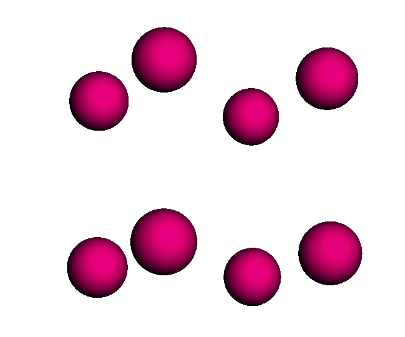Polonium simple cubic (SC) structure

### Body-centered Cubic (BCC)¶

• CsCl

import mbuild as mb
import numpy as np

# define all necessary lattice parameters
spacings = [0.4123, 0.4123, 0.4123]
angles = [90, 90, 90]
point1 = [[0, 0, 0]]
point2 = [[0.5, 0.5, 0.5]]

# define lattice object
bcc_lattice = mb.Lattice(lattice_spacing=spacings, angles=angles, lattice_points={'A' : point1, 'B' : point2})

# define Compounds
cl = mb.Compound(name='Cl')
cs = mb.Compound(name='Cs')

# populate lattice with compounds
cscl_lattice = bcc_lattice.populate(compound_dict={'A' : cl, 'B' : cs}, x=2, y=2, z=2)

# visualize
cscl_lattice.visualize()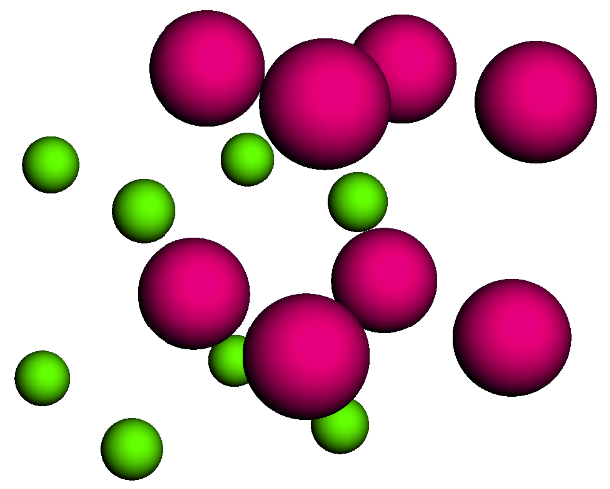CsCl body-centered cubic (BCC) structure

### Face-centered Cubic (FCC)¶

• Cu

import mbuild as mb
import numpy as np

# define all necessary lattice parameters
spacings = [0.36149, 0.36149, 0.36149]
angles = [90, 90, 90]
points = [[0, 0, 0], [0.5, 0.5, 0], [0.5, 0, 0.5], [0, 0.5, 0.5]]

# define lattice object
fcc_lattice = mb.Lattice(lattice_spacing=spacings, angles=angles, lattice_points={'A' : points})

# define Compound
cu = mb.Compound(name='Cu')

# populate lattice with compounds
cu_lattice = fcc_lattice.populate(compound_dict={'A' : cu}, x=2, y=2, z=2)

# visualize
cu_lattice.visualize()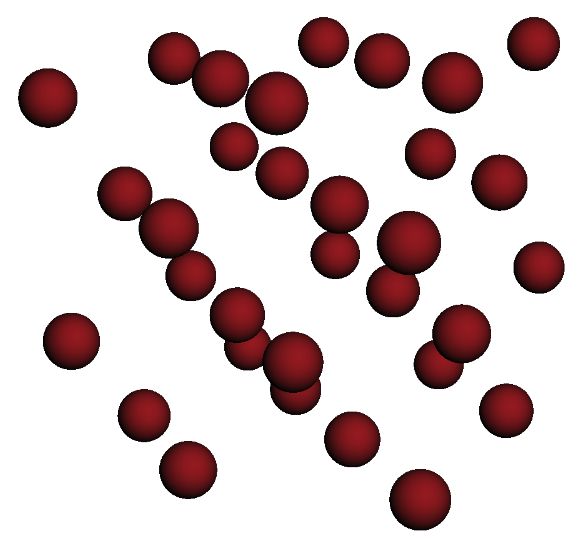Cu face-centered cubic (FCC) structure

### Diamond (Cubic)¶

• Si

import mbuild as mb
import numpy as np

# define all necessary lattice parameters
spacings = [0.54309, 0.54309, 0.54309]
angles = [90, 90, 90]
points = [[0, 0, 0], [0.5, 0.5, 0], [0.5, 0, 0.5], [0, 0.5, 0.5],
[0.25, 0.25, 0.75], [0.25, 0.75, 0.25], [0.75, 0.25, 0.25], [0.75, 0.75, 0.75]]

# define lattice object
diamond_lattice = mb.Lattice(lattice_spacing=spacings, angles=angles, lattice_points={'A' : points})

# define Compound
si = mb.Compound(name='Si')

# populate lattice with compounds
si_lattice = diamond_lattice.populate(compound_dict={'A' : si}, x=2, y=2, z=2)

# visualize
si_lattice.visualize()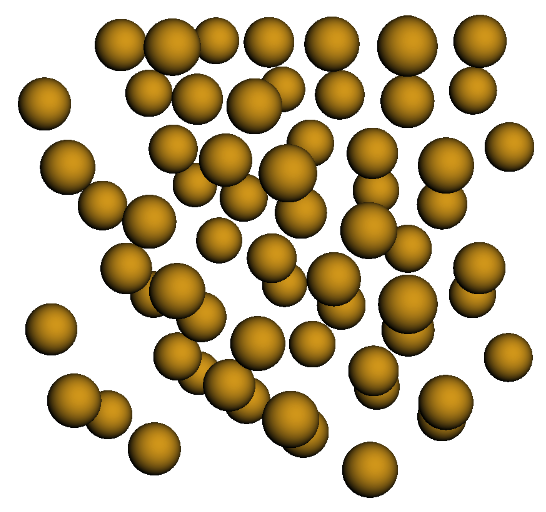Si diamond (Cubic) structure

### Graphene (2D)¶

• C

import mbuild as mb
import numpy as np

# define all necessary lattice parameters
spacings = [0.246, 0.246, 0.335]
angles = [90, 90, 120]
points = [[0, 0, 0], [1/3, 2/3, 0]]

# define lattice object
graphene_lattice = mb.Lattice(lattice_spacing=spacings, angles=angles, lattice_points={'A' : points})

# define Compound
c = mb.Compound(name='C')

# populate lattice with compounds
graphene = graphene_lattice.populate(compound_dict={'A' : c}, x=5, y=5, z=1)

# visualize
graphene.visualize()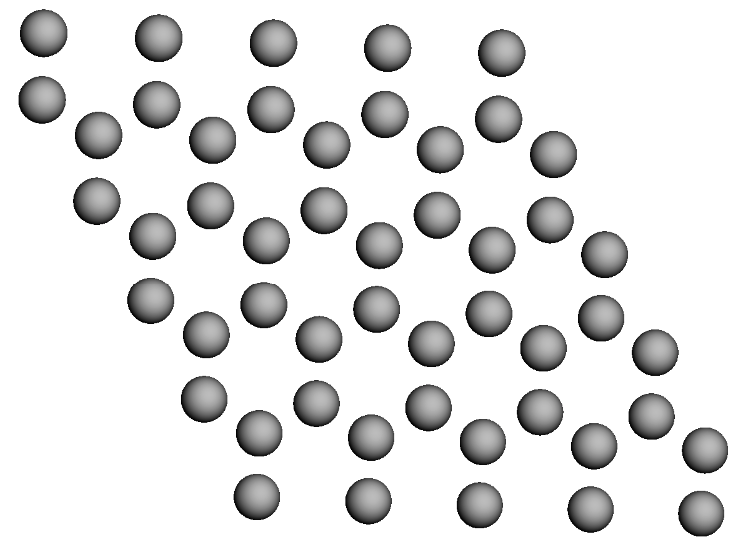Graphene (2D) structure